Net-Point Method

The following article is from The Great Soviet Encyclopedia (1979). It might be outdated or ideologically biased.

Net-Point Method

a translation of the Russian term metod setok, which is the collective name for a group of approximation methods of solving differential equations. With regard to partial differential equations, the term “net-point method” is used synonymously with the terms “method of finite differences” and “difference method,” which are terms commonly used in English. The net-point method is one of the most common approximation methods for solving problems involving differential equations. The extensive use of the method is due to its broad applicability and the comparative simplicity of its computer implementation.

The net-point method essentially involves replacing by a net—that is, by a discrete set of points, called vertices, or nodes—the range of continuous variation of arguments in which a solution of the equation is to be found; when necessary, the equation is supplemented with boundary and initial conditions. Instead of functions of a continuous argument, functions of a discrete argument, known as net functions, are considered; they are defined at the vertices of the net. Derivatives occurring in the equation and in the initial and boundary conditions are approximated by difference quotients, and integrals are approximated by quadrature formulas. In this case the initial equation (problem) is replaced by a system of algebraic equations, which is called a system of net equations; these equations are linear if the initial problem is linear. In the case of differential equations, the initial equation is replaced by a difference scheme.

If the system of net equations obtained in this manner is solvable—at least over a sufficiently fine net, that is, a net with densely packed vertices—and if, as the net is refined without bound, the solution of the system approaches, or converges to, the solution of the initial equation (problem), then the solution found over any fixed net is taken as the approximate solution of the initial equation (problem).

Let us consider the one-dimensional heat equation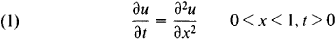with the initial condition μ(x, 0) = u0(x) and the boundary conditions u(0,t), = μ1(t), μ(1, t) = μ2 (t); it is assumed here that u0(0) = μ1(0) and u0(1) = μ2(0). In this case, when we have a rectangular uniform net with vertices (xi = ih, tj = jτ), where i = 0, 1,2,…, N, J = 0, 1, 2, …, A = l/N, and τ > 0 (h and τ describe the mesh size of the net), the most frequently used weighted difference scheme has the form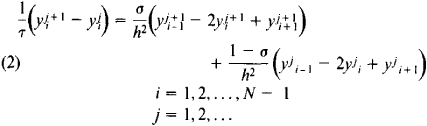Here, yji = y(xi, tj) and y0i = μ0 (xi)), i = 0, 1,2,…, N;y0j = μ1 (tj) and yN j = μ2 (tj). In this scheme, σ is some parameter. In the case of the two-dimensional Poisson equation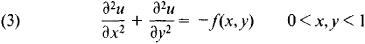with the homogeneous boundary conditions u(0, y) = u(x, 0) = u(1, y) = u(x, 1) = 0, over a uniform rectangular net with vertices

xi1=i1hi yi2=i2h2

where i1 = 0, 1, …, N1, i2 = 0, 1, …, N2, h1 = 1/N1, and h2 = 1/N2, the most frequently used scheme is the difference scheme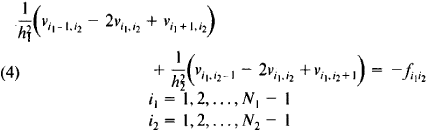Here,

v0,i 2 = vi 1,0 = vN1,i2 = vi1,N2 =0

i1 = 0, 1, …, N1

i2 = 0, 1, …, N1

In the case of the integral equation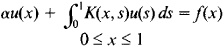the simplest system of net equations over a uniform net with vertices x, =ih, where i = 0, 1, 2,…, N and h = 1/N has the formIn addition to the uniform rectangular nets described above, nets of a more general form can be used—for example, nonuniform nets and, in the case of equation (3), nonrectangular nets. Over such nets the net equation assumes a more complex form. If equation (3) is solved in a nonrectangular region, the approximation of the boundary conditions becomes less self-evident, even over a uniform rectangular net.

In selecting a particular net-point approximation, the size of the approximation error is of great importance. Thus, in the case of equations (2), the approximation error is O(τ + h2) for any σ, O(τ2 + h2) for σ = 0.5, and O(τ2 + h4) for σ = 0.5 - h2/12τ. The approximation error for difference scheme (4) is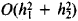. Even if the equations and boundary conditions are well approximated by the net equations, this does not guarantee that the solution of the system of net equations will in some sense be close to the solution of the initial problem. The solution of the net equations must be stable—that is, it must be a continuous (uniformly continuous with respect to the choice of the net) function of the right side and initial and boundary data. Thus, only when the approximation is good and the solution is stable is there any guarantee that the solutions of the net equations converge to the solution of the initial equation as the net is refined without limit. It should be noted that scheme (2) is stable when a ≥ 1/2 – h2/4τ; when σ = 0, an explicit scheme is obtained that is stable under the condition τ ≤ h2/2.

Systems of net equations are systems of linear algebraic equations. The finer the net, the higher the order of the system. The accuracy of the approximate solution, however, depends on the mesh size. The smaller the mesh size, the greater the accuracy. The algebraic systems obtained are therefore usually of a rather high order.

REFERENCES

Samarskii, A. A. Vvedenie v leoriiu raznostnykh skhem. Moscow, 1971.
Godunov, S. K. and V. S. Riaben’kii. Raznostnye skhemy. Moscow, 1973.

V. B. ANDREEV and A. A. SAMARSKII